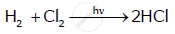# For a reaction Rate = k (i) Write the order and molecularity of this reaction. (ii) Write the unit of k. - Chemistry

For a reaction:Rate = k

(i) Write the order and molecularity of this reaction.

(ii) Write the unit of k.

#### Solution

(i) The reaction is a zero order reaction, and the molecularity of this reaction is 2.

(ii) The unit of the rate constant k is mole L−1 sec−1.

Concept: Factors Influencing Rate of a Reaction
Is there an error in this question or solution?